## Linearize the phase

### Quick description

When faced with the task of controlling an integral containing a phase such as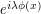, consider using a change of variables (or a Taylor-type approximation) to replace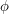with a simpler phase, such as a linear phase or a quadratic phase in normal form. This potentially allows one to use the tools of Fourier analysis or contour integration.

### Prerequisites

Harmonic analysis

### Example 1

Suppose one wants to show that a one-dimensional integral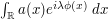is rapidly decreasing in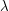, whereis a bump function andis a smooth phase which has no stationary points on the support of(and in particular, is strictly monotone on this support). Then one can perform a change of variables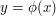to replace the integral with a Fourier integral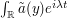, where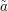is another bump function (which can be written explicitly in terms of, the change of variables function, and the Jacobian factor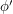). Since the Fourier transform of a bump function is rapidly decreasing, the claim follows.

Note that one can also establish this claim using "use integration by parts to exploit cancellation" or the method of stationary phase.

### Example 2

Now suppose one wants to understand the one-dimensional integral, wherenow has one stationary point on the support of, say at the origin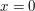. Suppose also thatis non-degenerate with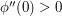. By Taylor expansion,near the origin. If we eliminate the contribution away from the origin (which is rapidly decreasing inby the previous example), and then perform a smooth change of variables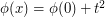, then one is faced with an integral of the form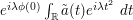, whereis a bump function with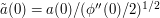. To proceed further, we create an epsilon of room and use the explicit integral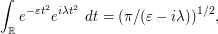(where we take a standard branch cut of the logarithm) which can be established by contour integration (or using the square and rearrange trick). One can then use "adding and subtracting" to write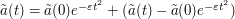. The contribution of the main term is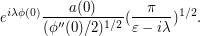The contribution of the error term is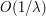. This can be seen by noting that one can pull a factor ofout of the amplitude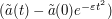, leaving a remainder which is still smooth; now we can "use integration by parts to exploit cancellation", taking advantage of the fact that we can antidifferentiate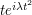. putting everything together, and letting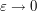, we obtain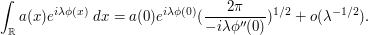In fact a full asymptotic expansion in powers of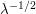can be obtaind by refining this method, leading to the method of stationary phase.

Note also that the asymptotics are consistent with base times height heuristics: the (signed) amplitude of the integrand at the stationary pointis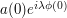, while the width of the interval where the phase is stationary (in the sense that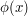only differs from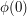byis about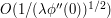, as can be seen from Taylor expansion.

### Example 3

(Talk about how wave packet decompositions are used to analyse FIOs, or to analyse the restriction problem)

### General discussion

In higher dimensions, placing a stationary phase in normal form may require Morse theory.